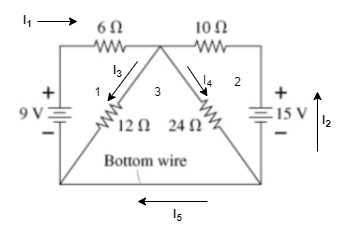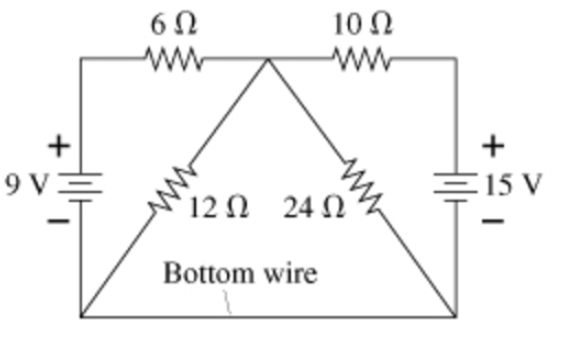# Problem: Part AHow much current flows through the bottom wire in the figure(Figure 1) ?Express your answer to two significant figures and include the appropriate units.Part BIn which direction current flows through the bottom wire in the figure?

###### FREE Expert Solution

Kirchhoff's voltage law:

$\overline{){\mathbf{\Sigma }}{\mathbf{V}}{\mathbf{=}}{\mathbf{0}}}$

From Ohm's law:

$\overline{){\mathbf{V}}{\mathbf{=}}{\mathbf{I}}{\mathbf{R}}}$, where I is current and R is resistance.

Let's label the different regions in the diagram as shown below:Part A:

Consider loop 1 in a clockwise direction:

9 - 6I1 - 12I3 = 0

99% (4 ratings)###### Problem Details

Part A

How much current flows through the bottom wire in the figure(Figure 1) ?

Express your answer to two significant figures and include the appropriate units.

Part B

In which direction current flows through the bottom wire in the figure?